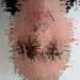## Interview Question – BCD Digit, Multiplied by 5

December 21, 2008

A while back, someone sent me the interview question I am about to describe, asking for help. I think it serves a very good example of observing patterns and not rushing into conclusions.
I will immediately post the answer after describing the problem. However, I urge you to try and solve it on your own and see what you came up with. On we go with the question…

Design a circuit with minimum logic that receives a single digit, coded BCD (4 wires) and as an output gives you the result multiplied by 5 – also BCD coded (8 wires).

So, I hope you got a solution ready at hand and you didn’t cheat 😉 .

Let’s first make some order and present the input and required outputs in a table (always a good habit).Looking for some patterns we can see that we actually don’t need any logic at all to solve this problem!!You will be amazed how many people get stuck with a certain solution and believe it is the minimal one. Especially when the outcome is one or two single gates. When you tell them it can be done with less, they will easily find the solution. IMHO there is nothing really clever or sophisticated about this problem, but it demonstrates beautifully how it is sometimes hard for us to escape our initial ideas and approaches about a problem.

Coming to think of it, this post was more about psychology and problem solving than digital design – please forgive…

1.This question was put to me @ infineon couple yrs back 🙂

2.and… did you fall in the trap or you saw past it?

3.I actually came up with a simple mathematical proof to the result

4.nice Q.[:)]

5.5*(8a3+4a2+2a1+a0)
=40a3+20a2+10a1+5a0
=40a3+20a2+10a1+4a0+a0

maps to

80b7+40b6+20b5+10b4 + 8b3+4b2+2b1+b0

if b6==a3, b5==a2, b4==a1, b2==a0 and b0=a0

6.We have to apply huge logic here to solve this.

7.Isn’t decimal 10 is 1010 and not 10000 – which would invalidate the whole design – since the second LSB will not be always 0?

•No. I guess, in BCD coded system each digit is coded independently. Kindly correct me if am wrong.

8.module test(a,b);
input [3:0]a;
output [7:0]b;
wire [3:0]a;
reg [3:0]temp;
reg [7:0] b;

assign #1 a = 4’b0110;

initial
begin
#1
temp = a;
temp = 0;
b[7:4] = temp>>1;
if(a)
b[3:0] = 4’b0101;
else
b[3:0] = 4’b0000;
\$display(“The value of input a is %h”, a);
\$display(“The value of output b is %h”, b);
end
endmodule

9.No gates are required to solve this problem
we can see that all even no have lsb=0 and odd have lsb=1 and for even the lower 4 bits are 0000 and for odd the lower 4 bits are 0101.Thus to generate the lower 4 bis just supply LSB of given no to bit position 2 and 4.This gives lower for bits.
To genertae upper 4 bits msb=0 always and just supply the 1st 3 bits of the number.

10.i am B.tech student, i dont know how to do ” BCD digit multiply by another BCD digit”, pls can u show me the complete hardware ( logic gates)

11.It can be sloved like this
for 4 bit input a3a2a1a0
8bit output will be b7b6b5b4b3b2b1b0
output bits will be related to input as
b7 = 0
b6 = a3
b5 = a2
b4 = a1
b3 = 0
b2 = a0
b1 = 0
b0 = a0

12.modulo 10 multiplier (shift 5 times to right ) and then use modulo 2 divider

13.if (a)
y = {a[3:1], 4’b0101};
else
y = {a[3:1],4’b0000};